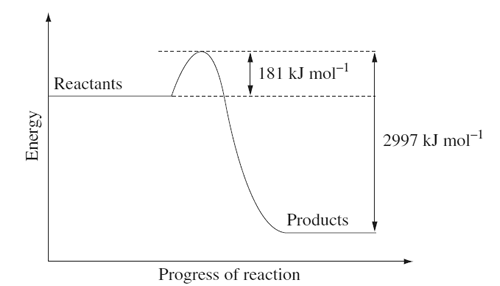Thermo-chemistry (2009 NSW HSC) 1) Calculate the mass of ethanol that must be burnt to increase the temperature of 210 g of water by 65°C, if exactly half of the heat released by this combustion is lost to the surroundings. The heat of combustion of ethanol is 1367 kJ mol−1 . Solution 2) What are TWO ways to limit heat loss from the apparatus when performing a first-hand investigation to determine and compare heat of combustion of different liquid alkanols? Solution3) The following energy diagram shows the energy change associated with the combustion of glucose in kJ mol–1 during cellular respiration.(i) Write a balanced chemical equation for the combustion of glucose. Include the energy released per mole of glucose. Solution (ii)Calculate the energy released per gram of glucose. Solution (iii) An average cyclist uses 80 kJ for every km travelled. If the glucose content of an energy bar is 36.7 g, how far can an average cyclist travel on only one energy bar? Solution